# ICSE Class 10 Physics Question Paper Solution 2019

Solving the ICSE class 10 physics question paper questions gives students ideas on types of questions asked in the exam. Furthermore it also helps in understanding the difficulty level of the exam. Students get to know the proportion of numerical and theoretical questions asked in the Class 10 Physics board exam. So, to help students, we have provided the ICSE Class 10 Physics Question Paper Solution 2019. Here, students will find the detailed answer of each question.

The ICSE Class 10 Physics 2019 exam was conducted on 5th March 2019. The exam started at 11am and students were allotted 2 hours of time duration to finish the paper. Students can download the ICSE Class 10 Physics Question Paper Solution 2019 PDF from the link below.

Students can have a look at the ICSE Class 10 Physics Question Paper Solution 2019 below: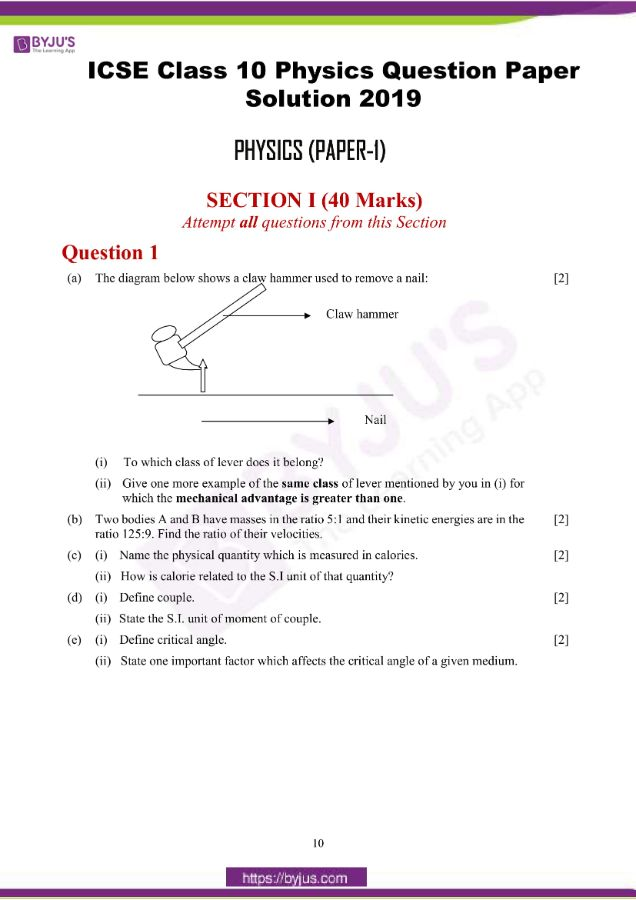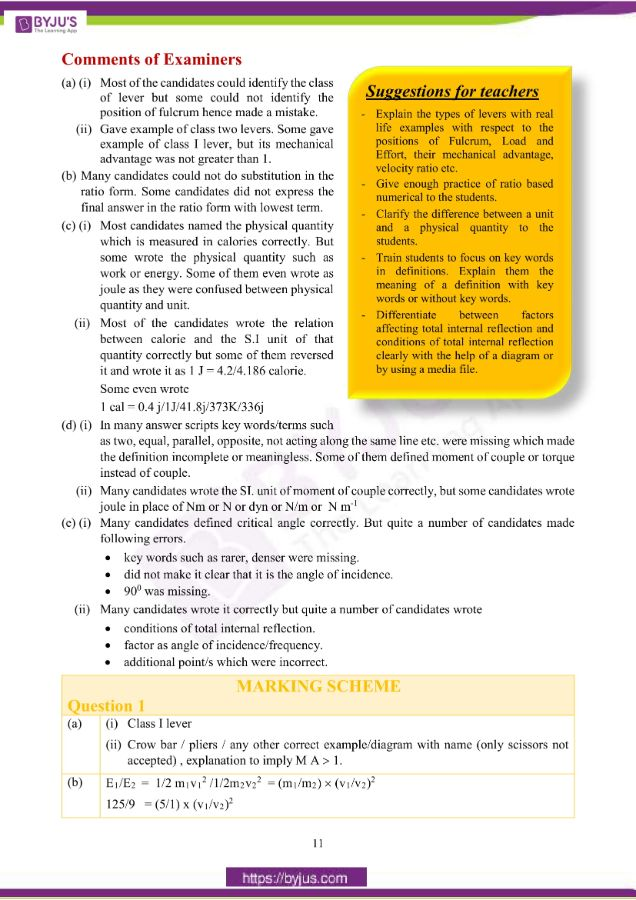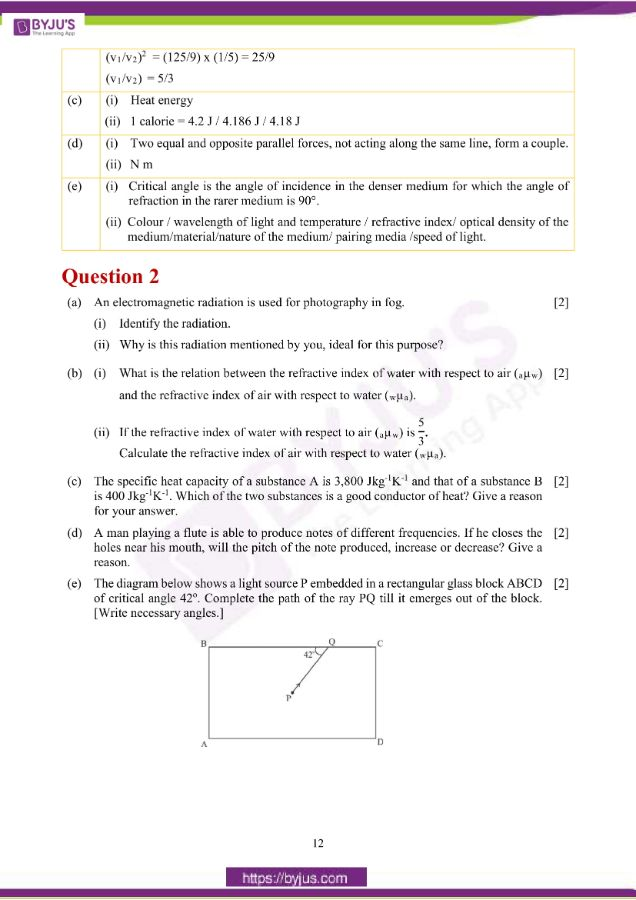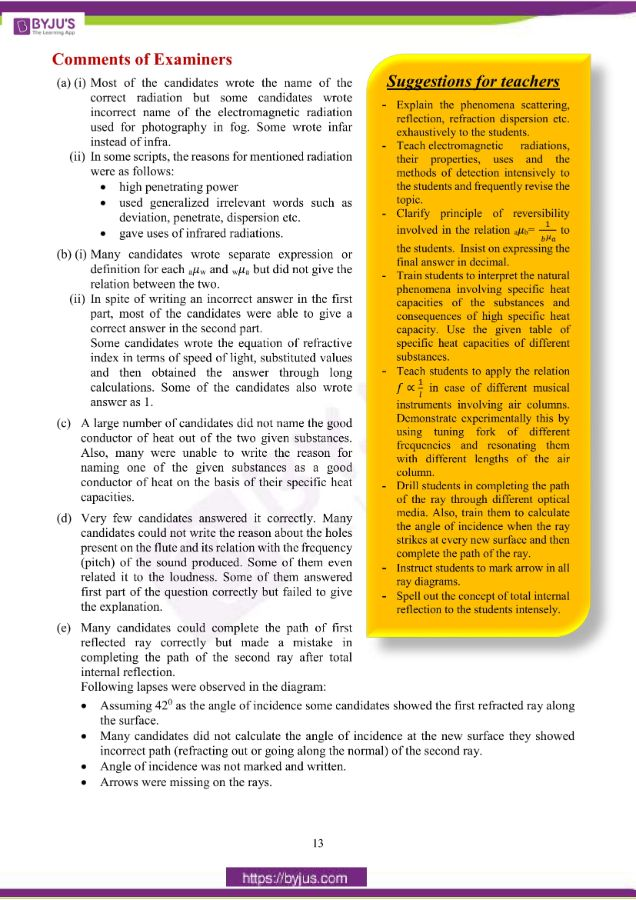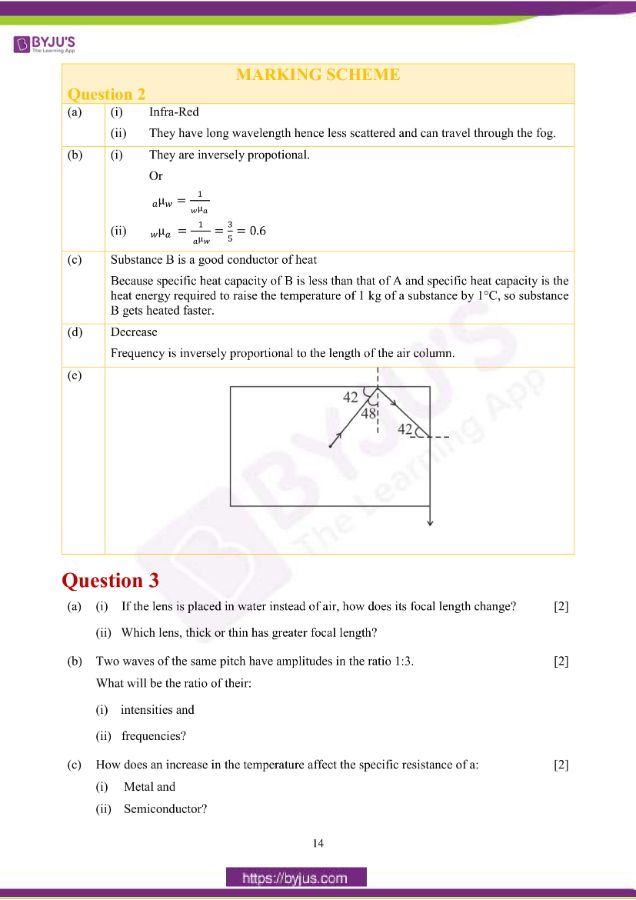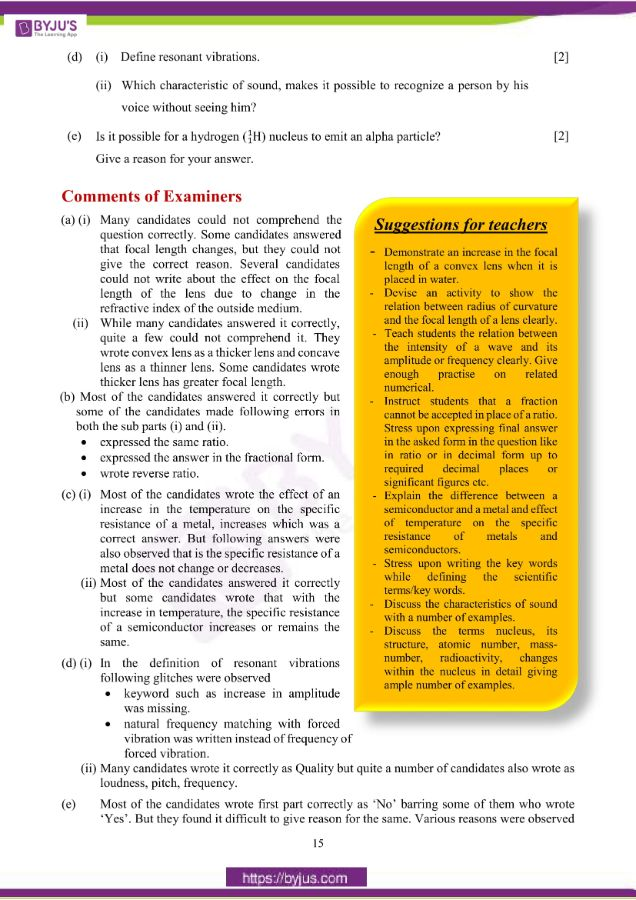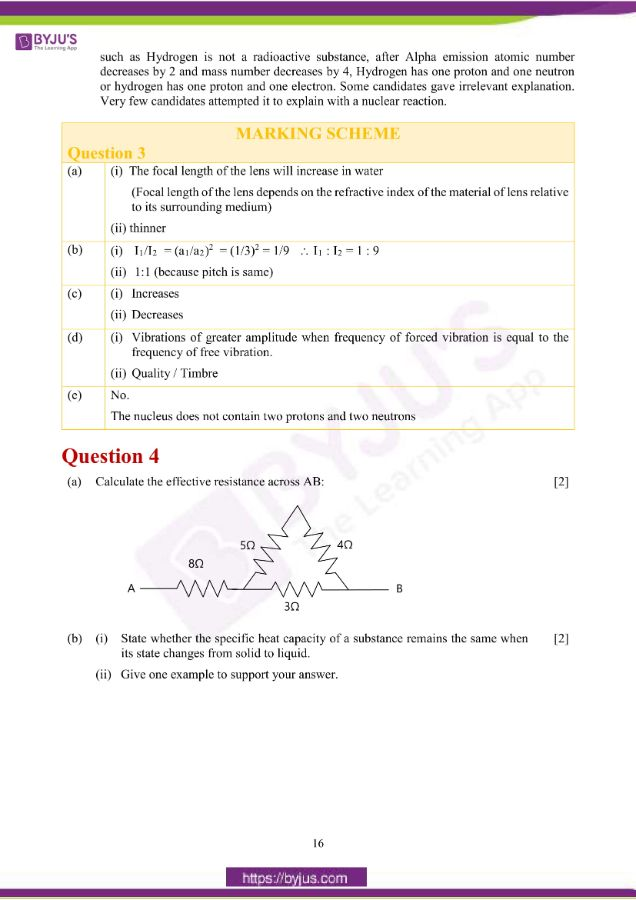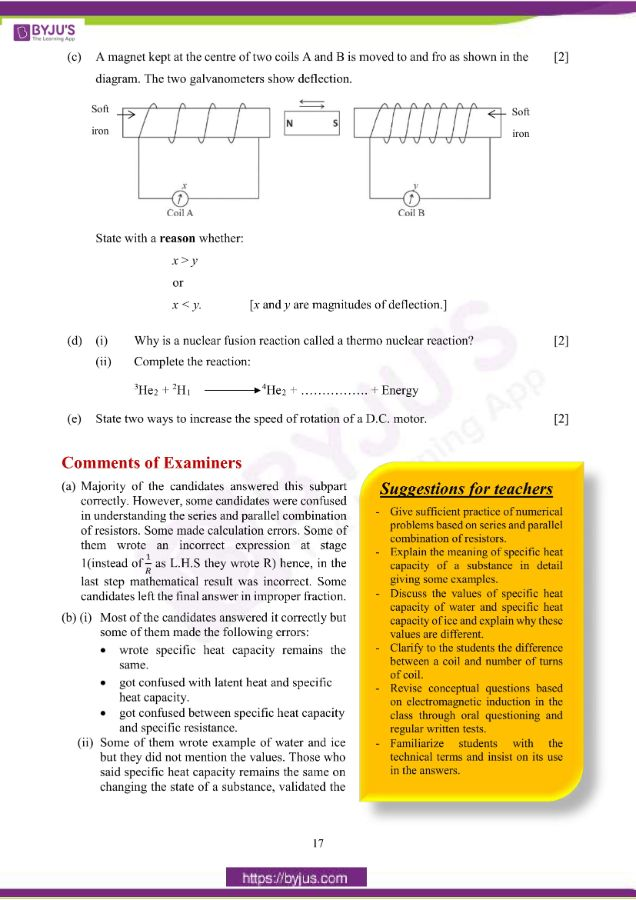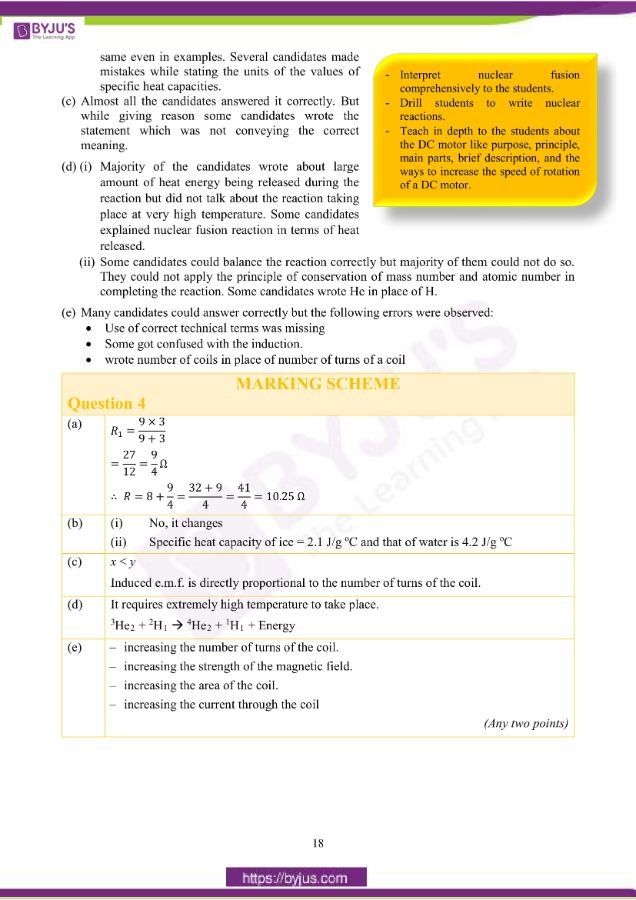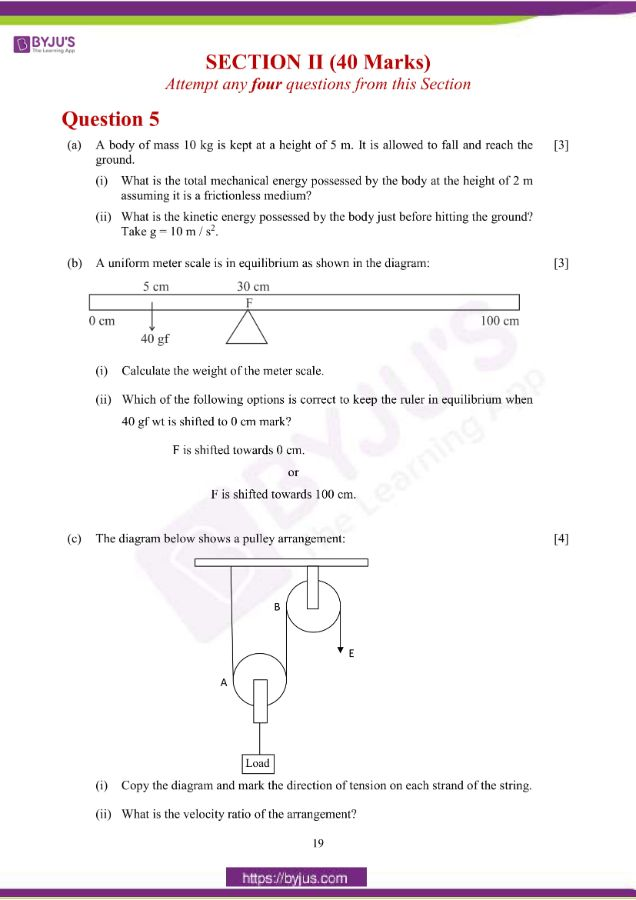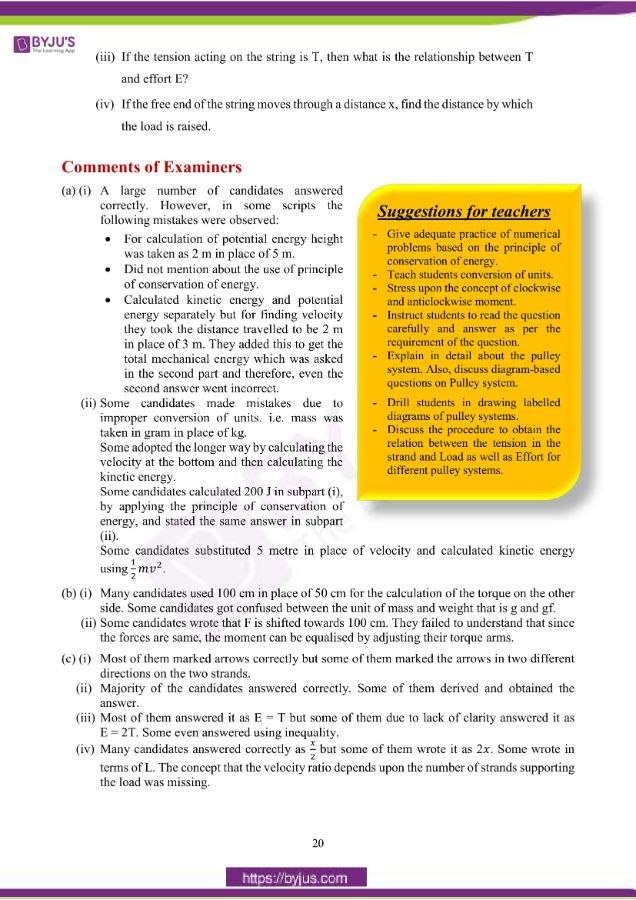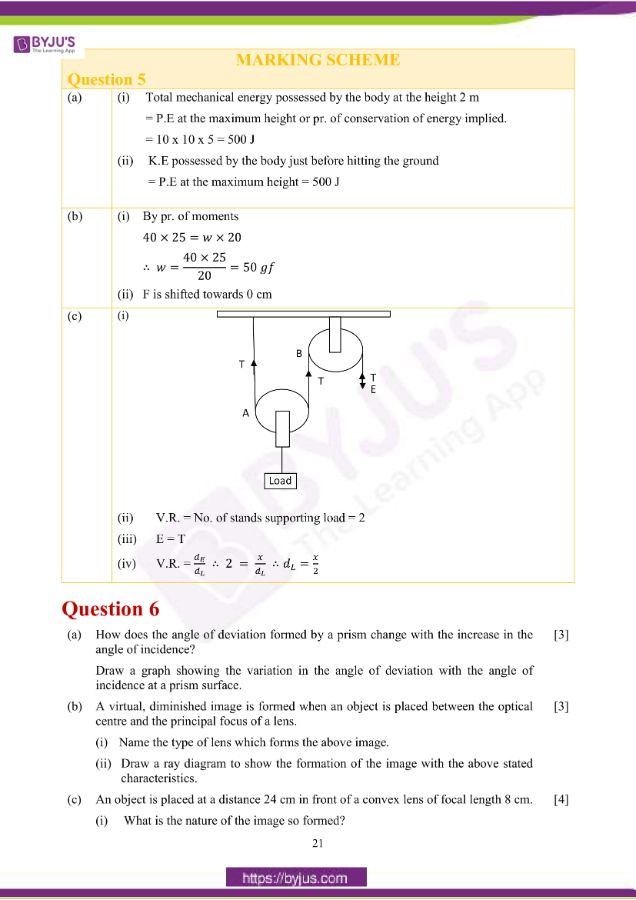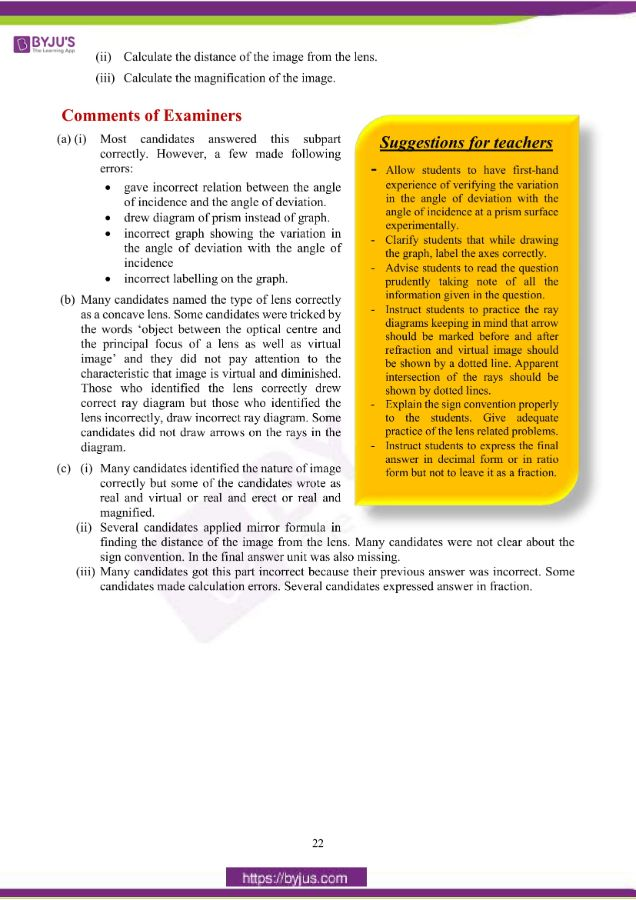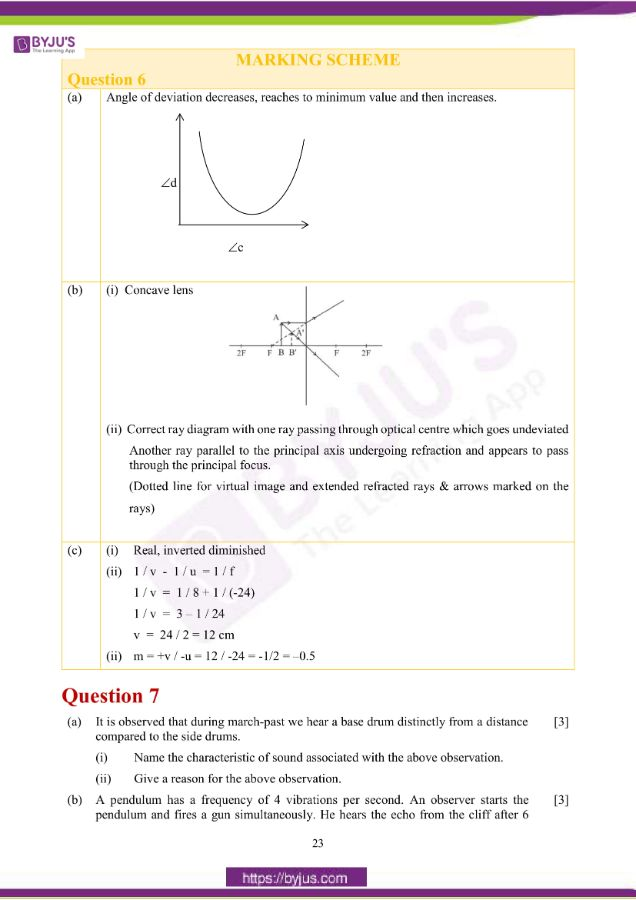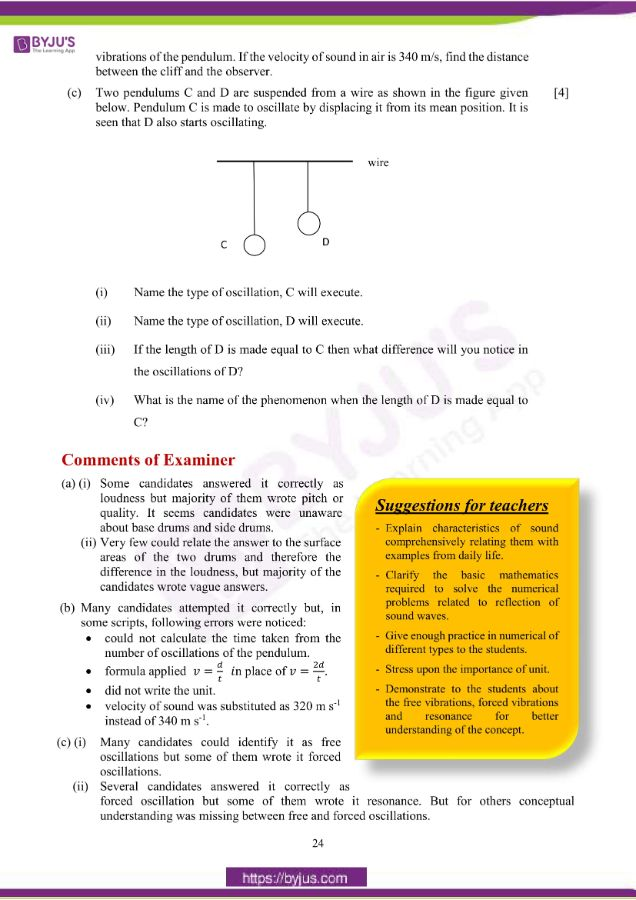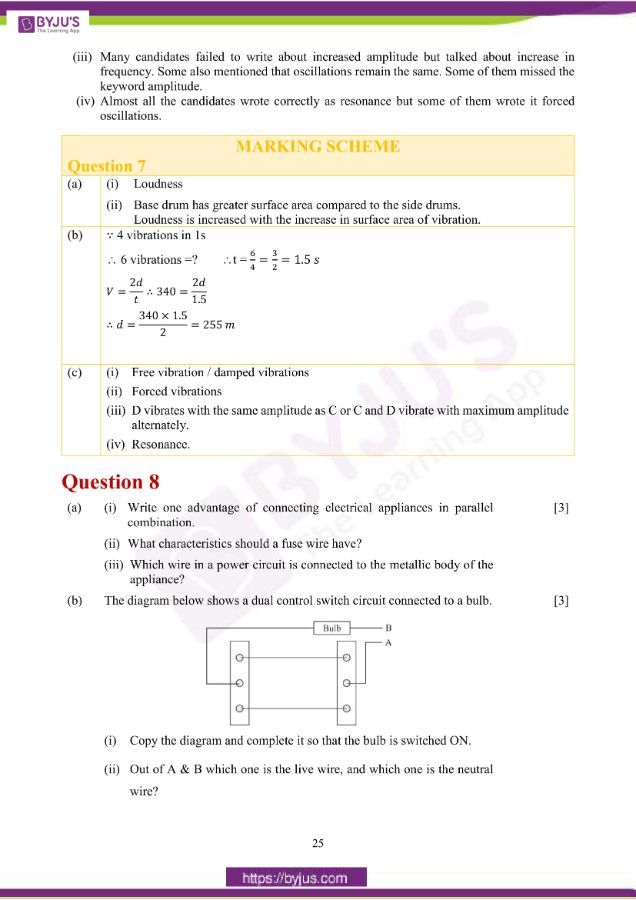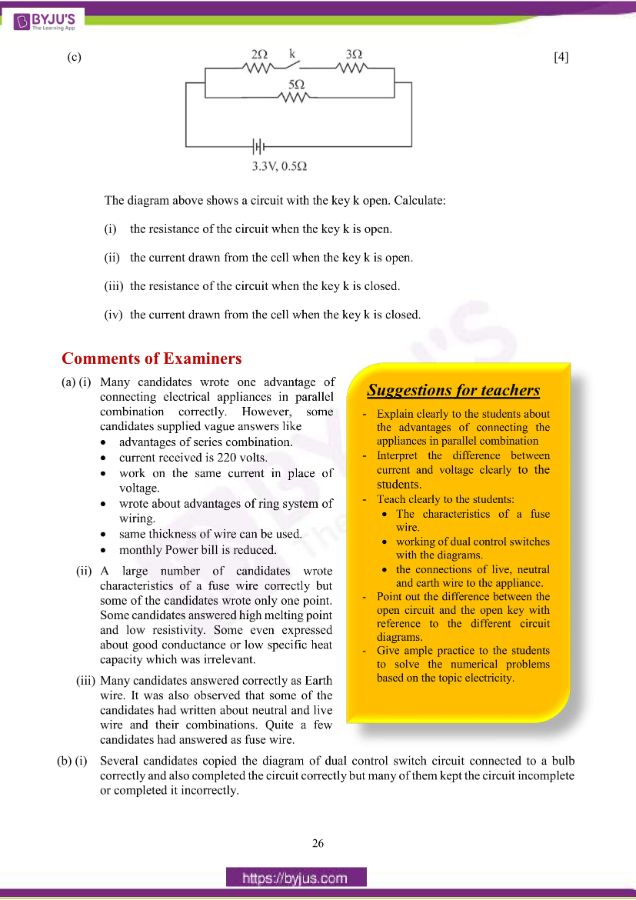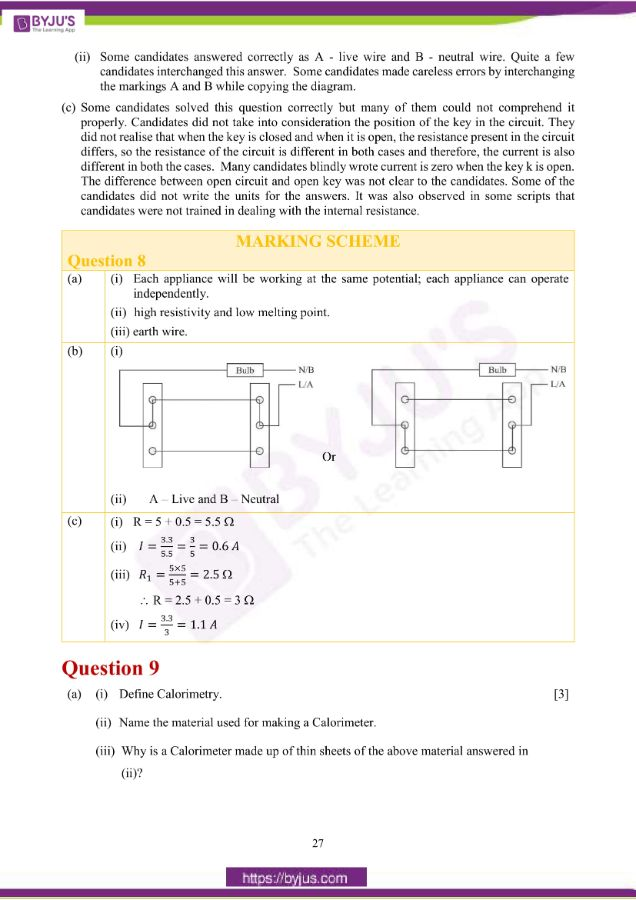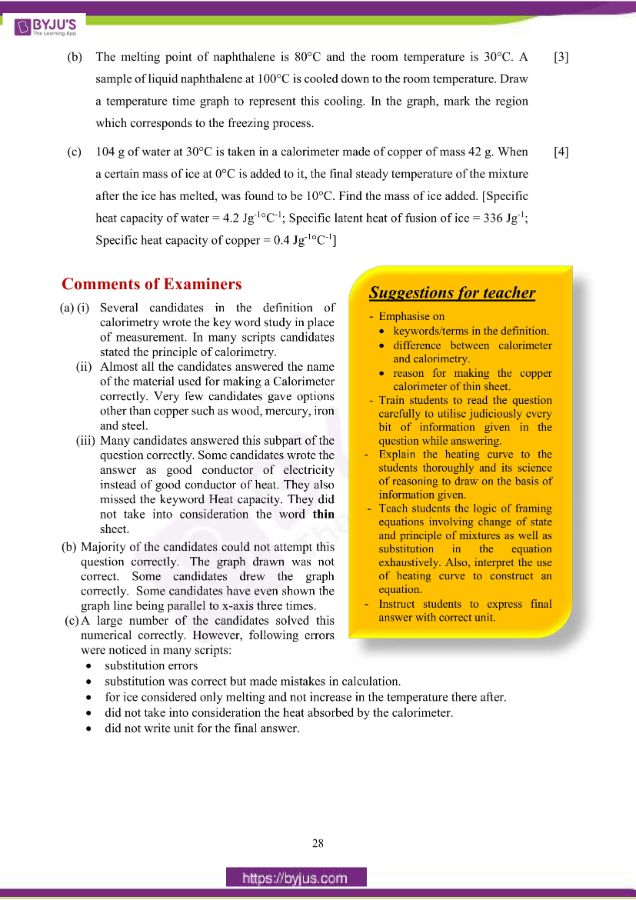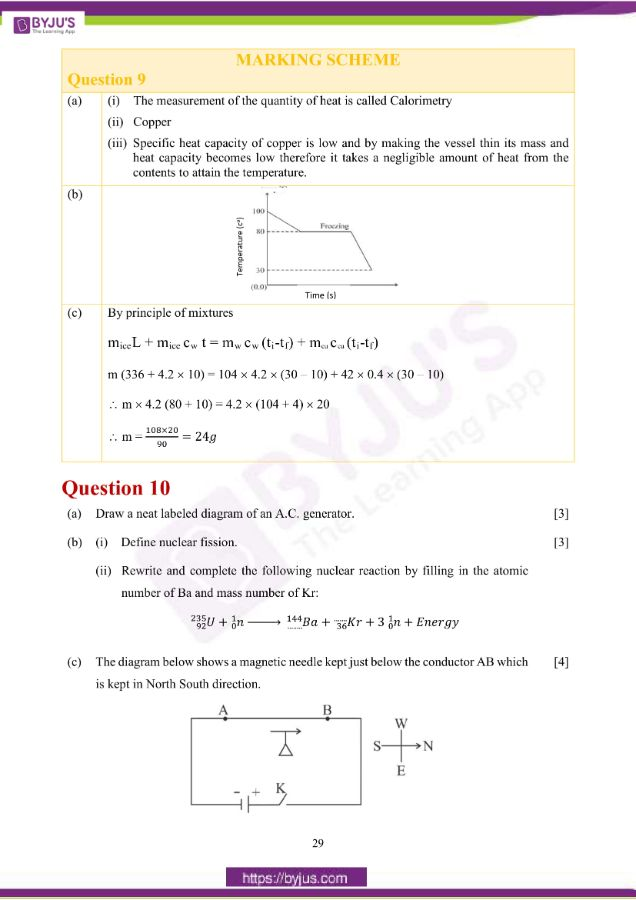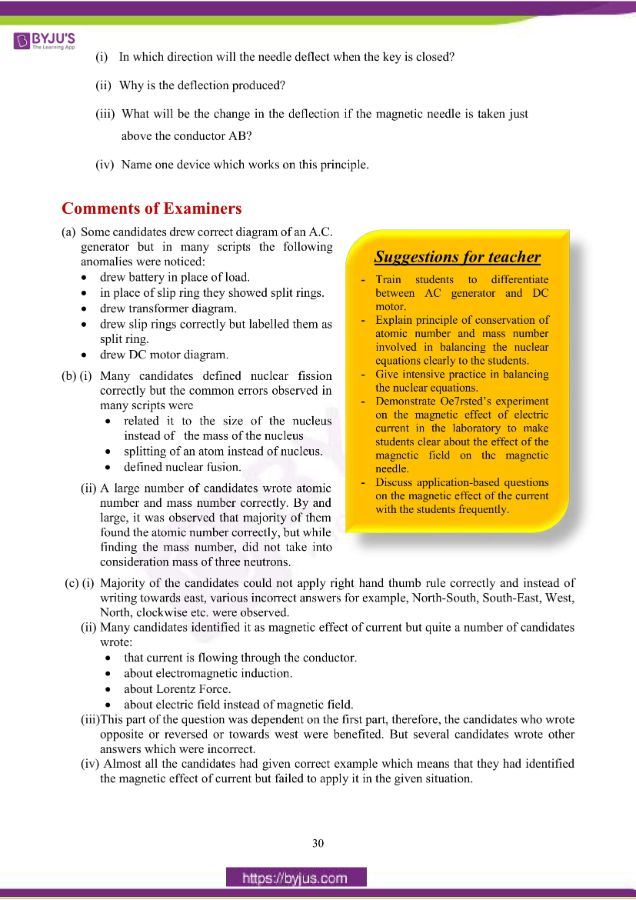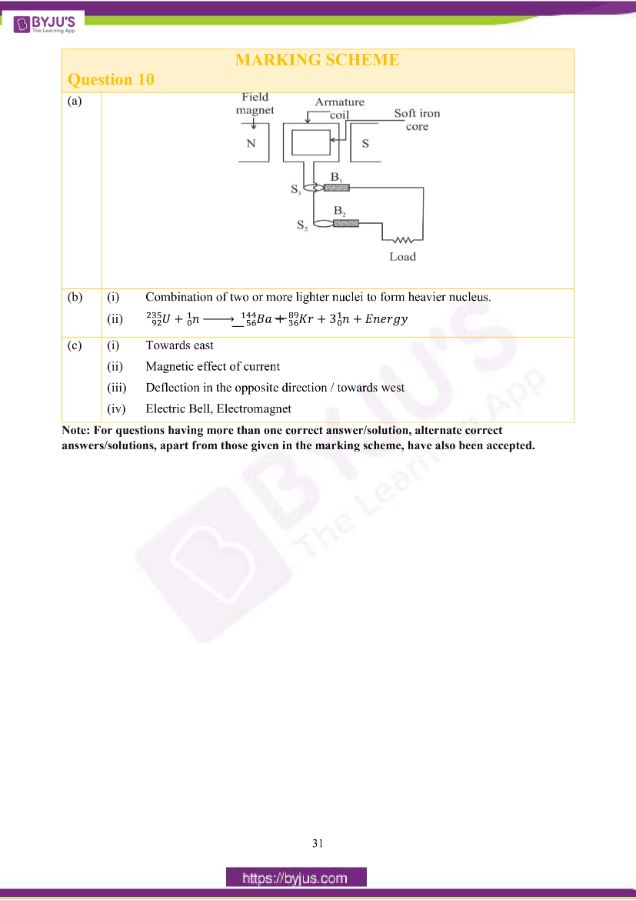## ICSE Class 10 Physics Question Paper 2019 With Solution

### Question 1:

(a) The diagram below shows a claw hammer used to remove a nail: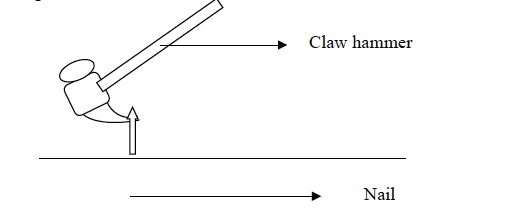(i) To which class of lever does it belong?

(ii) Give one more example of the same class of lever mentioned by you in (i) for

which the mechanical advantage is greater than one.

(b) Two bodies A and B have masses in the ratio 5:1 and their kinetic energies are in the ratio 125:9. Find the ratio of their velocities.

(c) (i) Name the physical quantity which is measured in calories.

(ii) How is calorie related to the S.I unit of that quantity?

(d) (i) Define couple.

(ii) State the S.I. unit of moment of couple.

(e) (i) Define critical angle.

(ii) State one important factor which affects the critical angle of a given medium.

(a) (i) Class I lever

(ii) Crow bar / pliers / any other correct example/diagram with name (only scissors not

accepted) , explanation to imply M A > 1.

(b)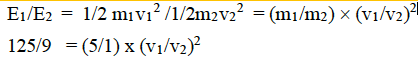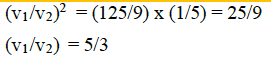(c) (i) Heat energy

(ii) 1 calorie = 4.2 J / 4.186 J / 4.18 J

(d) (i) Two equal and opposite parallel forces, not acting along the same line, form a couple.

(ii) N m

(e) (i) Critical angle is the angle of incidence in the denser medium for which the angle of

refraction in the rarer medium is 90°.

(ii) Colour / wavelength of light and temperature / refractive index/ optical density of the

medium/material/nature of the medium/ pairing media /speed of light.

### Question 2:

(a) An electromagnetic radiation is used for photography in fog.

(ii) Why is this radiation mentioned by you, ideal for this purpose?

(b) (i) What is the relation between the refractive index of water with respect to air (aμw) and the refractive index of air with respect to water (wμa).

(ii) If the refractive index of water with respect to air (aμw) is

5/3. Calculate the refractive index of air with respect to water (wμa).

(c) The specific heat capacity of a substance A is 3,800 Jkg-1K-1 and that of a substance B is 400 Jkg-1K-1. Which of the two substances is a good conductor of heat? Give a reason for your answer.

(d) A man playing a flute is able to produce notes of different frequencies. If he closes the holes near his mouth, will the pitch of the note produced, increase or decrease? Give a reason.

(e) The diagram below shows a light source P embedded in a rectangular glass block ABCD of critical angle 42o. Complete the path of the ray PQ till it emerges out of the block.

[Write necessary angles.]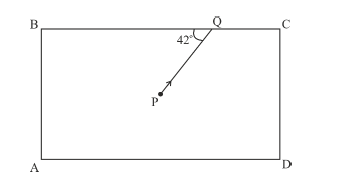(a) (i) Infra-Red

(ii) They have long wavelength hence less scattered and can travel through the fog.

(b) (i) They are inversely proportional.

Or

𝑤 = 1/ 𝑤μ𝑎

(ii) wμ𝑎 = 1/𝑎μ𝑤= 3/5 = 0.6

(c) Substance B is a good conductor of heat

Because specific heat capacity of B is less than that of A and specific heat capacity is the heat energy required to raise the temperature of 1 kg of a substance by 1°C, so substance B gets heated faster.

(d) Decrease

Frequency is inversely proportional to the length of the air column.

(e)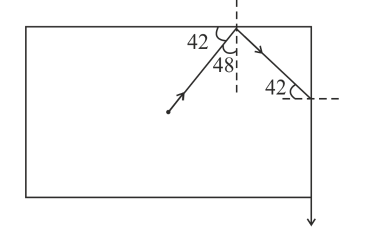### Question 3:

(a) (i) If the lens is placed in water instead of air, how does its focal length change?

(ii) Which lens, thick or thin has greater focal length?

(b) Two waves of the same pitch have amplitudes in the ratio 1:3.

What will be the ratio of their:

(i) intensities and

(ii) frequencies?

(c) How does an increase in the temperature affect the specific resistance of a:

(i) Metal and

(ii) Semiconductor?

(d) (i) Define resonant vibrations.

(ii) Which characteristic of sound, makes it possible to recognize a person by his

voice without seeing him?

(e) Is it possible for a hydrogen ( 11H) nucleus to emit an alpha particle? Give a reason for your answer.

(a) (i) The focal length of the lens will increase in water

(Focal length of the lens depends on the refractive index of the material of lens relative to its surrounding medium)

(ii) thinner

(b) (i) I1/I2 = (a1/a2)2 = (1/3)2 = 1/9

∴ I1 : I2 = 1 : 9

(ii) 1:1 (because pitch is same)

(c) (i) Increases

(ii) Decreases

(d) (i) Vibrations of greater amplitude when frequency of forced vibration is equal to the

frequency of free vibration.

(ii) Quality / Timbre

(e) No.

The nucleus does not contain two protons and two neutrons

### Question 4:

(a) Calculate the effective resistance across AB: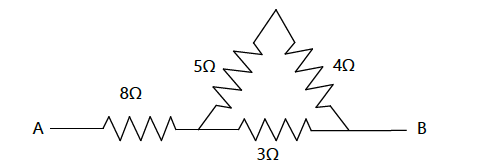(b) (i) State whether the specific heat capacity of a substance remains the same when its state changes from solid to liquid.

(c) A magnet kept at the centre of two coils A and B is moved to and fro as shown in the

diagram. The two galvanometers show deflection.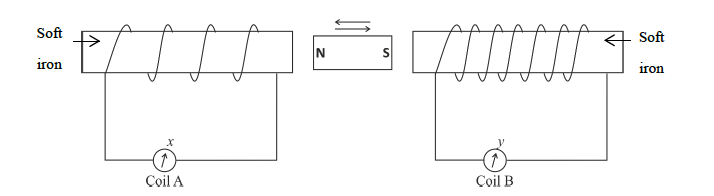State with a reason whether:

x > y

or

x < y. [x and y are magnitudes of deflection.]

(d) (i) Why is a nuclear fusion reaction called a thermo nuclear reaction?

(ii) Complete the reaction:

3He2 + 2H14He2 + ……………. + Energy

(e) State two ways to increase the speed of rotation of a D.C. motor.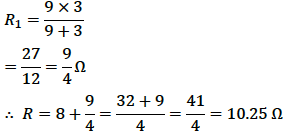(b) (i) No, it changes

(ii) Specific heat capacity of ice = 2.1 J/g oC and that of water is 4.2 J/g oC

(c) x < y

Induced e.m.f. is directly proportional to the number of turns of the coil.

(d) It requires extremely high temperature to take place.

3He2 + 2H14He2 + 1H1 + Energy

(e) – increasing the number of turns of the coil.

– increasing the strength of the magnetic field.

– increasing the area of the coil.

– increasing the current through the coil

### Question 5:

(a) A body of mass 10 kg is kept at a height of 5 m. It is allowed to fall and reach the ground.

(i) What is the total mechanical energy possessed by the body at the height of 2 m assuming it is a frictionless medium?

(ii) What is the kinetic energy possessed by the body just before hitting the ground?

Take g = 10m / s2.

(b) A uniform meter scale is in equilibrium as shown in the diagram: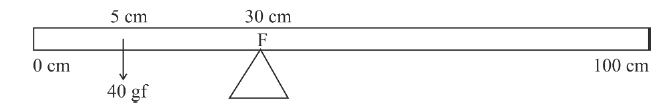(i) Calculate the weight of the meter scale.

(ii) Which of the following options is correct to keep the ruler in equilibrium when

40 gf wt is shifted to 0 cm mark?

F is shifted towards 0 cm.

or

F is shifted towards 100 cm.

(c) The diagram below shows a pulley arrangement:

(i) Copy the diagram and mark the direction of tension on each strand of the string.

(ii) What is the velocity ratio of the arrangement?

(i) Copy the diagram and mark the direction of tension on each strand of the string.

(ii) What is the velocity ratio of the arrangement?

(i) Copy the diagram and mark the direction of tension on each strand of the string.

(ii) What is the velocity ratio of the arrangement?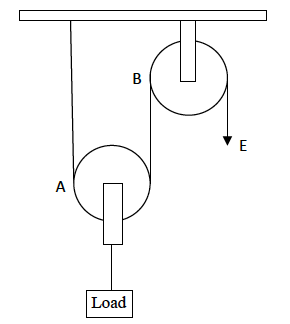(i) Copy the diagram and mark the direction of tension on each strand of the string.

(ii) What is the velocity ratio of the arrangement?

(iii) If the tension acting on the string is T, then what is the relationship between T

and effort E?

(iv) If the free end of the string moves through a distance x, find the distance by which

(a) (i) Total mechanical energy possessed by the body at the height 2 m

= P.E at the maximum height or pr. of conservation of energy implied.

= 10 x 10 x 5 = 500 J

(ii) K.E possessed by the body just before hitting the ground

= P.E at the maximum height = 500 J

b) (i) By pr. of moments

40 × 25 = 𝑤 × 20

∴ 𝑤 = 40 × 25 / 20 = 50𝑔𝑓

(ii) F is shifted towards 0 cm

(c) (i)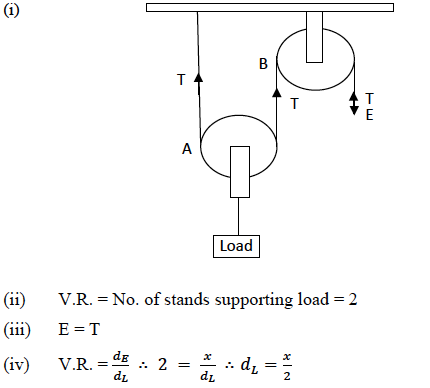### Question 6:

(a) How does the angle of deviation formed by a prism change with the increase in the

angle of incidence?

Draw a graph showing the variation in the angle of deviation with the angle of

incidence at a prism surface.

(b) A virtual, diminished image is formed when an object is placed between the optical

centre and the principal focus of a lens.

(i) Name the type of lens which forms the above image.

(ii) Draw a ray diagram to show the formation of the image with the above stated

characteristics.

(c) An object is placed at a distance 24 cm in front of a convex lens of focal length 8 cm.

(i) What is the nature of the image so formed?

(ii) Calculate the distance of the image from the lens.

(iii) Calculate the magnification of the image.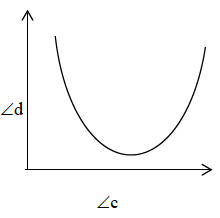(b) (i) Concave lens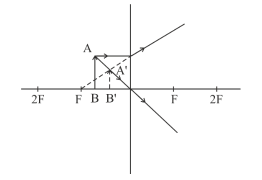(ii) Correct ray diagram with one ray passing through the optical centre which goes undeviated Another ray parallel to the principal axis undergoing refraction and appears to pass through the principal focus.

(Dotted line for virtual image and extended refracted rays & arrows marked on the

rays).

(c)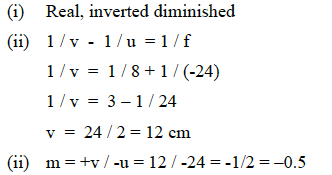### Question 7:

(a) It is observed that during march-past we hear a base drum distinctly from a distance compared to the side drums.

(i) Name the characteristic of sound associated with the above observation.

(ii) Give a reason for the above observation.

(b) A pendulum has a frequency of 4 vibrations per second. An observer starts the pendulum and fires a gun simultaneously. He hears the echo from the cliff after 6 vibrations of the pendulum. If the velocity of sound in air is 340 m/s, find the distance between the cliff and the observer.

(c) Two pendulums C and D are suspended from a wire as shown in the figure given below. Pendulum C is made to oscillate by displacing it from its mean position. It is seen that D also starts oscillating.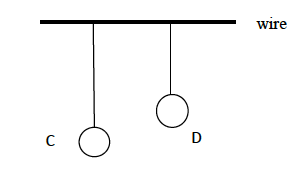(i) Name the type of oscillation, C will execute.

(ii) Name the type of oscillation, D will execute.

(iii) If the length of D is made equal to C then what difference will you notice in the oscillations of D?

(iv) What is the name of the phenomenon when the length of D is made equal to C?

(a) (i) Loudness

(ii) Base drum has greater surface area compared to the side drums.

Loudness is increased with the increase in surface area of vibration.

(b)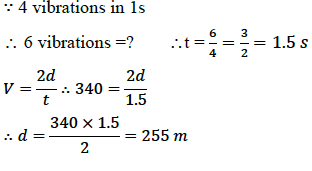(c) (i) Free vibration / damped vibrations

(ii) Forced vibrations

(iii) D vibrates with the same amplitude as C or C and D vibrate with maximum amplitude

alternately.

(iv) Resonance.

### Question 8:

(a) (i) Write one advantage of connecting electrical appliances in parallel combination.

(ii) What characteristics should a fuse wire have?

(iii) Which wire in a power circuit is connected to the metallic body of the appliance?

(b) The diagram below shows a dual control switch circuit connected to a bulb.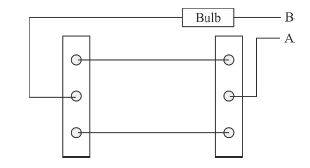(i) Copy the diagram and complete it so that the bulb is switched ON.

(ii) Out of A & B which one is the live wire, and which one is the neutral

wire?

(c)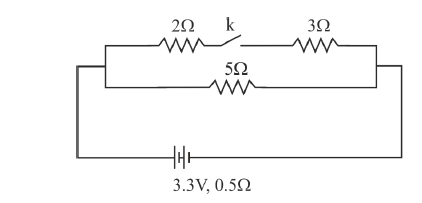The diagram above shows a circuit with the key k open. Calculate:

(i) the resistance of the circuit when the key k is open.

(ii) the current drawn from the cell when the key k is open.

(iii) the resistance of the circuit when the key k is closed.

(iv) the current drawn from the cell when the key k is closed.

(a) (i) Each appliance will be working at the same potential; each appliance can operate independently.

(ii) high resistivity and low melting point.

(iii) earth wire.

(b)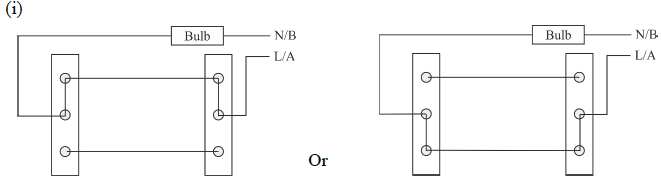(ii) A – Live and B – Neutral

(c)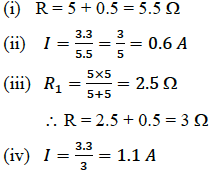### Question 9:

(a) (i) Define Calorimetry.

(ii) Name the material used for making a Calorimeter.

(iii) Why is a Calorimeter made up of thin sheets of the above material answered in (ii)?

(b) The melting point of naphthalene is 80°C and the room temperature is 30°C. A sample of liquid naphthalene at 100°C is cooled down to room temperature. Draw a temperature time graph to represent this cooling. In the graph, mark the region which corresponds to the freezing process.

(c) 104 g of water at 30°C is taken in a calorimeter made of copper of mass 42 g. When a certain mass of ice at 0°C is added to it, the final steady temperature of the mixture after the ice has melted, was found to be 10°C. Find the mass of ice added. [Specific heat capacity of water = 4.2 Jg-1°C-1; Specific latent heat of fusion of ice = 336 Jg-1; Specific heat capacity of copper = 0.4 Jg-1°C-1]

(a) (i) The measurement of the quantity of heat is called Calorimetry

(ii) Copper

(iii) Specific heat capacity of copper is low and by making the vessel thin its mass and heat capacity becomes low therefore it takes a negligible amount of heat from the contents to attain the temperature.

(b)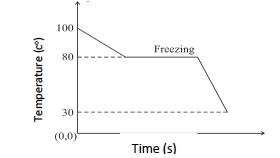(c)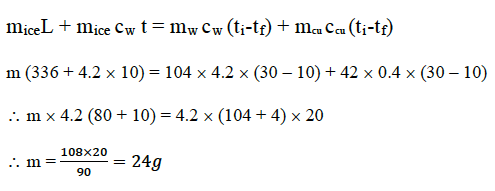### Question 10:

(a) Draw a neat labeled diagram of an A.C. generator.

(b) (i) Define nuclear fission.

(ii) Rewrite and complete the following nuclear reaction by filling in the atomic number of Ba and mass number of Kr: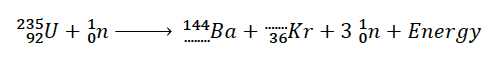(c) The diagram below shows a magnetic needle kept just below the conductor AB which is kept in the North South direction.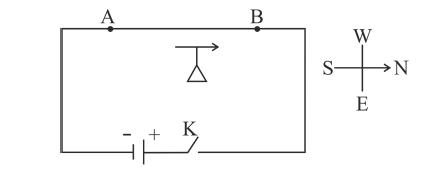(i) In which direction will the needle deflect when the key is closed?

(ii) Why is the deflection produced?

(iii) What will be the change in the deflection if the magnetic needle is taken just above the conductor AB?

(iv) Name one device which works on this principle.

(a)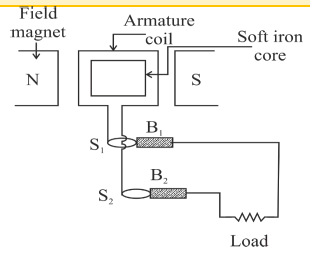(b) (i) Combination of two or more lighter nuclei to form heavier nucleus.

(ii)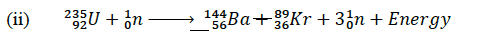(c) (i) Towards east

(ii) Magnetic effect of current

(iii) Deflection in the opposite direction / towards west

(iv) Electric Bell, Electromagnet

We hope this information on “ICSE Class 10 Physics Question Papers Solutions 2019” helped students in their exam preparation. To get the year wise ICSE Class 10 Previous Years Question papers along with solutions for other subjects, click here. Keep learning and download BYJU’S App to access interactive study videos.